# Surds and Indices - Quantitative Aptitude (MCQ) questions

Dear Readers, Welcome to Quantitative Aptitude Surds and Indices questions and answers with explanation. These Surds and Indices solved examples with shortcuts and tricks will help you learn and practice for your Placement Test and competitive exams like Bank PO, IBPS PO, SBI PO, RRB PO, RBI Assistant, LIC,SSC, MBA - MAT, XAT, CAT, NMAT, UPSC, NET etc.

After practicing these tricky Surds and Indices multiple choice questions, you will be exam ready to deal with any objective type questions.

1)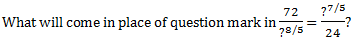- Published on 03 May 17

a. 12
b. 6
c. 16
d. 8
 Answer  Explanation ANSWER: 12 Explanation: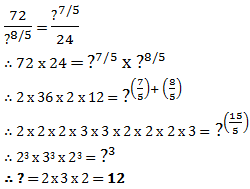2)   What is value of (0.000001) ?
- Published on 03 May 17

a. 0.000001
b. 0.001
c. 1/100
d. 0.1
 Answer  Explanation ANSWER: 1/100 Explanation: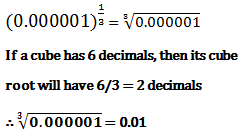3)   What will be value of 46.7856 if, (684)2= 467856?
- Published on 03 May 17

a. 6.84
b. 0.0684
c. 0.684
d. 0.000684
 Answer  Explanation ANSWER: 6.84 Explanation: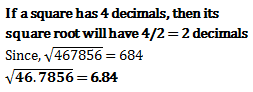4)   If value of 28 is approximately 5.2915, then value of 7/4 is approximately
- Published on 03 May 17

a. 1.2000
b. 0.5687
c. 1.3228
d. 1.4652
 Answer  Explanation ANSWER: 1.3228 Explanation: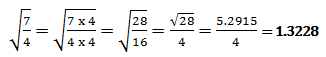5)   How many zeroes are there in 200010?
- Published on 03 May 17

a. 10
b. 30
c. 20
d. 15
 Answer  Explanation ANSWER: 30 Explanation: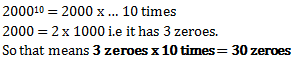6)   What is value of (36)?
- Published on 03 May 17

a. 2
b. 36
c. 1
d. 36
 Answer  Explanation ANSWER: 36 Explanation: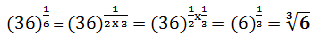7)   What is value of M in (p/q)2M+2 = (q/p)9-M
- Published on 13 Apr 17

a. 6
b. 5
c. -7/2
d. -11
 Answer  Explanation ANSWER: -11 Explanation: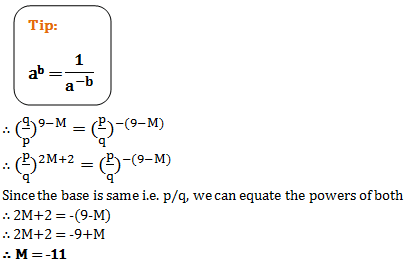8)   What is value of 22(-2)
- Published on 12 Apr 17

a. -16
b. -42
c. 42
d. 1/16
 Answer  Explanation ANSWER: 42 Explanation: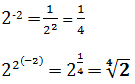9)   What is value of 2(-2)2
- Published on 12 Apr 17

a. 1/16
b. 1/8
c. 32
d. 16
 Answer  Explanation ANSWER: 16 Explanation: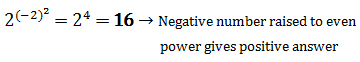10)   There are four expressions given below separated by ‘=’ sign. Which one of the 4 expressions is not the same as the other three?
62 x 6 = (61)3 = 23+33 = 83 - 142 - 102

- Published on 11 Apr 17

a. (61)3
b. 83 - 142 - 102
c. 23+33
d. All are same
 Answer  Explanation ANSWER: 23+33 Explanation: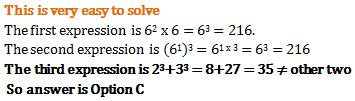1 2